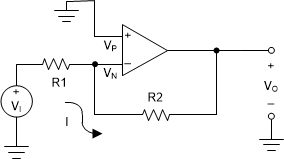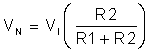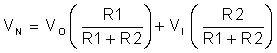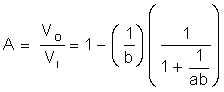SLOA011B January   2018  – July 2021

1. 1Introduction
2. 2Non-Inverting Amplifier
3. 3Inverting Amplifier
4. 4Simplified Op Amp Circuit Diagram
5. 5Op Amp Specifications
6. 6References
7. 7Glossary
8. 8Revision History

# 3 Inverting Amplifier

Figure 3-1 shows another useful basic op amp circuit, the inverting amplifier. The triangular gain block symbol is again used to represent an ideal op amp. The input terminal, + (Vp), is called the non-inverting input, whereas – (Vn) marks the inverting input. It is similar to the non-inverting circuit shown in Figure 2-1 except that now the signal is applied to the inverting terminal via R1 and the non-inverting terminal is grounded.Figure 3-1 Inverting Amplifier

To understand this circuit, we must derive a relationship between the input voltage, Vi and the output voltage, Vo.

Since Vp is tied to ground,

Equation 21. Vp = 0

Remembering that there is no current into the input, the voltage at Vn can be found using superposition. First let Vo = 0,

Equation 22.Next let Vi = 0,

Equation 23.Combining

Equation 24.Remembering equation Equation 14, Vo = aVd = a(Vp - Vn), substituting and rearranging,

Equation 25.where

Equation 26.Again we have an amplifier circuit. Because b ≤ 1, the closed loop gain, A, is negative, and the polarity of Vo will be opposite to Vi. Therefore, this is an inverting amplifier.